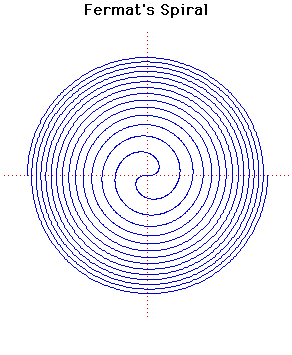# Curves

### Fermat's SpiralPolar equation:
$r^{2} = a^{2} \theta$

### Description

This spiral was discussed by Fermat in 1636.

For any given positive value of θ there are two corresponding values of $r$, one being the negative of the other. The resulting spiral will therefore be symmetrical about the line$y = -x$ as can be seen from the curve displayed above.

The inverse of Fermat's Spiral, when the pole is taken as the centre of inversion, is the spiral $r^{2} = a^{2}/ \theta$.

For technical reasons with the plotting routines, when evolutes, involutes, inverses and pedals are drawn only one of the two branches of the spiral are drawn.

### Associated Curves

Definitions of the Associated curves